Differential manifold

(Redirected from Differentiable manifold)

Definition

If this is the first time you're seeing the definition of a differential manifold, you may want to explore the definition more carefully in the survey article How we define manifolds and why

Definition in terms of atlases

A differential manifold or smooth manifold is the following data:

• A topological manifold$M$ (in particular,$M$ is Hausdorff and second-countable)
• An atlas of coordinate charts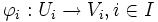$\varphi_i:U_i \to V_i, i \in I$ from$M$ to$\R^n$ (in other words an open cover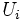$U_i$ of$M$ with homeomorphisms from each member$U_i$ of the open cover to open sets$V_i$ in$\R^n$)

satisfying the compatibility condition: the transition function between any two coordinate charts of the atlas is a diffeomomorphism of open subsets of$\R^n$. In symbols: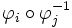$\varphi_i \circ \varphi_j^{-1}$

is a homeomorphism when restricted to the set: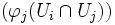$(\varphi_j(U_i \cap U_j))$

By diffeomorphism, we here mean a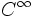$C^{\infty}$ map with a$C^{\infty}$ inverse.

However, we need to quotient out this data by the following equivalence:

Two atlases of coordinate charts on a topological space define the same differential manifold structure if taking their union still gives an atlas of coordinate charts. In other words, given any coordinate chart in one atlas and any coordinate chart in the other atlas, the transition function between them is a diffeomorphism.

Definition in terms of sheaves

A differential manifold or smooth manifold is the following data:

• A topological manifold$M$
• A subsheaf of the sheaf of continuous functions from$M$ to$\R$, which plays the role of the sheaf of infinitely differentiable functions

Such that for every$p \in M$, there exists an open set$U \ni p$ and a homeomorphism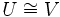$U \cong V$ where$V$ is an open subset of$\R^n$, such that the sheaf restricted to$U$ corresponds, via the homeomorphism, to the usual sheaf of infinitely differentiable functions on$V$.

Examples and properties

Occurrence of differential manifolds

Differential manifolds occur in many different situations. The simplest example of a differential manifold is an open subset of Euclidean space: here, the coordinate chart is simply its inclusion into Euclidean space.

More generally, differential manifolds include structures that locally look like Euclidean space, though they may have global properties that prevent them from being embeddable as open subsets of Euclidean space. For instance, a sphere is a differential manifold that can be given an atlas with two coordinate charts: the two open subsets are the complements of the north and south pole respectively, and the homeomorphisms to Euclidean space are stereographic projections.

Viewpoints of the collection of differential manifolds

Differential manifolds are often viewed as a category, where the morphisms are smooth maps. For more information, refer Category of differential manifolds with smooth maps.

Constructions

Sheaf of infinitely differentiable functions

One way of viewing the definition of a differential manifold is that it provides criteria on what functions can be viewed as differentiable, and what functions cannot be viewed as differentiable. All this data is captured in the sheaf of infinitely differentiable functions. This sheaf associates, to every open subset, a subalgebra of the algebra of continuous functions on that subset, which are deemed to be infinitely differentiable.